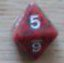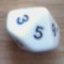Different/Highest 2dx-2dx + Dice Step Open Ended Roll

## Different/Highest 2dx-2dx + Dice Step Open Ended Roll

Return to topic list

 Sat Feb 1 11:30:13 2014   by   RobMuadib HelloI was working on another variation of my +0 average dice mechanic and am wondering if I am doing it right. MECHANIC CONCEPTI wanted a system where attributes affect your basic success curve, with a gradual increase per point. Implemented as a dice-step system allowing it to handle large attribute values without massive quantities of dice. So you have base 2dx-2dx and attributes add a dice to positive dice pool if pos, negative dice pool if neg. Instead of straight die per point, I wanted to use a die step system. You add a d4/d6/d8/d10/d12 to your dice pool for +/- 1,2,3,4,5 repeat. Adding a die every 5 points of Stat instead of +1. To go with intuitive feel of adding progressively larger die roll, base mechanic on counting different dice. MECHANIC DESCRIPTIONDICE POOLSYou have two dice pools A positive dice pool, consisting of a base 2d6. And a negative dice pool also consisting of a base 2d6 die. Your Attribute Score adds dice to these dicepools based on it's dice step.  If your attribute score is positive, you add one or more bonus dice to your positive dice poolIf your attribute score is negative you add one or more penalty dice to your negative dice pool.DICE STEPSThe Dice Step corresponding to a Stats Rank Score represents the size, and number of dice you add to the base dice pool of 1d10. The positive dice pool if the Stat Score is positive, and the negative dice pool if the Stat Score is negative. Note you don't add any dice to either dice pool. The Dice Steps Chart below shows the Dice Step corresponding to each Rank Score.Dice StepDice-10  -2d12-9  -1d12+d10-8  -1d12+d8)-7  -1d12+d6-6  -1d12+d4-5  -d12)-4  -d10)-3  -d8-2  -d6-1  -d4+0  + d0+1  +d4+2  +d6+3  +d8+4  +d10+5  +d12+6  +d12+d4+7  +d12+d6+8  +d12+d8+9  +d12+d10+10  +2d12MAXED ROLLSAfter rolling your dice, examine the results of each dice pool, positive and negative, individually.  Look for "Maxed Rolls", that is a die on which the highest number possible has been thrown. So, 4 on a d4, 6 on a d6, 8 on a d8, 10 on a d10, 12 on a d12. For each MAXED ROLL you roll and add another die, of the same type, to the corresponding dice pool. For instance, a positive d4 would spawn a positive d4, while a negative d10 would spawn a negative d10. Roll and add another die of the same type, adding it to its respective dice pool. If that die throw is a MAXED ROLL, you can roll and add another die of the same type to the corresponding dice pool, again . You continue rolling and adding dice, of the corresponding size, to each respective dice pool as long you keep throwing MAXED ROLLS.DICE RESULTS  (SETS and BANDS)After having rolled the dice and rerolled any Maxed Rolls, you determine the result of each dice pool. First combine the dice in each pool into matching sets. That is, dice with the same number thrown.  If there is only 1 of a particular number it is a set of 1, called an Orphan Set. The number of different sets is referred to as your Range Band. The more different sets, the higher the Range Band of your result. Range Band  Sets  Result0            1    Zero1            2    Highest Number Thrown2            3    5+ Highest Number Thrown3            4    10 + Highest Number Thrown4            5    15 + Highest Number Thrown+1          +1    +5 + highest Number thrown.If All the dice thrown in a dice pool match (There are none different), it is a range band of zero, and the result is zero.  If there are two sets of matching dice, it is Range Band 1, the result is equal to the highest number thrown on the dice.  If there are three sets of matching dice it is range band 2, and the result is equal to 5 plus the highest number thrown on the dice. If there are four sets of matching dice, it is range band 3 and the result is equal to 10 + highest number thrown.  If there are five sets of matching dice, it is range band 4 and the result is equal to 15 plus the highest number thrown.  And so on.TROLL CODEHere is what I came up with, a variation of earlier code.I am not sure it is right however, as it produces a a double spiked symmetric curve with spikes at +0 and +5 When I am thinking it should be smoothly symmetric. \ Match Different/Highest 2d-2d +Die Step Mechanicfunction ppool(n,m,o) =  roll := n#(accumulate x:=d6 while x=6) U m#(accumulate x:=d4 while x=4) U o#(accumulate x:=d12 while x=12);  if (count different roll) = 0 \ all the same = RB 0  then roll = 0  else if (count different roll) = 1 \ 2 different RB 1    then max roll  else (max roll) + (((count different roll)-1)*5)\ 3 different RB 2function npool(n,m,o) =  roll := n#(accumulate x:=d6 while x=6) U m#(accumulate x:=d4 while x=4) U o#(accumulate x:=d12 while x=12);  if (count different roll) = 0 \ all the same  then roll = 0  else if (count different roll) = 1 \ 2 different RB 1    then max roll  else (max roll) + (((count different roll)-1)*5)\ 3 different RB 2P := 2;N := 2;call ppool(2,1,0) - call npool(2,0,0)Thank you for your assistance.Rob Sat Feb 1 18:33:34 2014   by   RobMuadib worked on the Troll code some more, think I have it right now, as it is behaving as I expected. This is a nicely behaving dice mechanic, used the count different dice idea you mentioned in your rpgdice paper. TROLL CODE\ Match Different/Highest 2d-2d +Die Step Mechanicfunction ppool(n,m,o) =  roll := n#(accumulate x:=d10 while x=10) U m#(accumulate x:=d8 while x=8) U o#(accumulate x:=d12 while x=12);  if (count different roll) = 0 \ all the same   then roll = 0  else if (count different roll) = 1 \ 2 different RB 1    then max roll  else max (foreach i in roll do ((count i = roll)-1)*5+i)function npool(n,m,o) =  roll := n#(accumulate x:=d10 while x=10) U m#(accumulate x:=d4 while x=4) U o#(accumulate x:=d12 while x=12);  if (count different roll) = 0 \ all the same   then roll = 0  else if (count different roll) = 1 \ 2 different RB 1    then max roll  else max (foreach i in roll do ((count i = roll)-1)*5+i)call ppool(2,0,2) - call npool(2,0,0) Sat Feb 1 20:54:49 2014   by   Rob Muadib Ok, after some sleep and working more with the mechanic I realized I coded that all wrong, color me embarrassed. The rerolls are strictly bound to 1 more per die (any more just adds matches to a set, which I was going to use special results.) So you max out based on the number and size of spawning dice minus a 0 result (all the same). Which can achieve a pretty high result with 4 dice in your pool and really lucky open-ended results. like +24 from a 2d6+d12+d4 pool. Would have to think on it more to code something proper.

Return to topic list

New message:
 Topic: Posted by:

Type the values of the dice shown below:Return to topic list